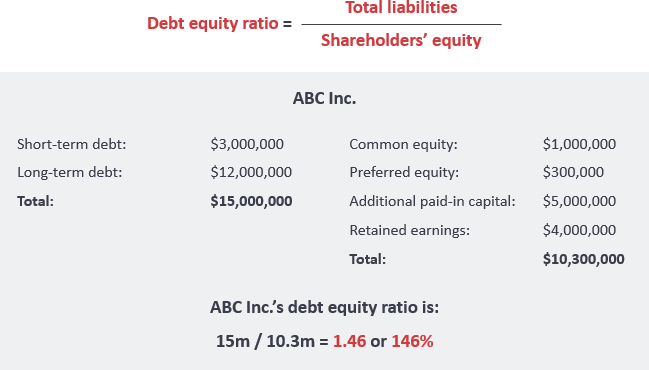# Debt to Equity Ratio Calculator

This is an online debt to equity ratio calculator.The debt-to-equity ratio (D/E) is a financial ratio indicating the relative proportion of shareholders’ equity and debt used to finance a company’s assets. In other words, it is calculated by dividing a company’s total liabilities by its shareholder equity. These figures are available on the balance sheet of a company’s financial statements.

## Debt to Equity Ratio Formula

In a general sense, the ratio is simply debt divided by equity. However, what is classified as debt can differ depending on the interpretation used.

Thus, the ratio can take on a number of forms including:

• Debt / Equity
• Long-term Debt / Equity
• Total Liabilities / Equity

Where

Total liabilities – Total liabilities represent all of a company’s debt, including short-term and long-term debt, and other liabilities (e.g., loans, overdrafts, and deferred tax liabilities).

Shareholders’ equity – Shareholders’ equity is found in the balance sheet and includes amounts invested by the owners and any retained profits.

## Example of ABC Company## Debt to Equity Ratio Calculators

#### This calculator is created with

the best online spreadsheet editor with excellent formula and editing capability.

For more calculators for finance, mathematics health, unit converters and more check out our calculators collection.